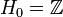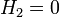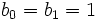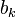# Klein bottle

## Definition

The Klein bottle is a compact non-orientable surface (and hence, in particular, a connected two-dimensional manifold) defined in the following equivalent ways (up to homeomorphism)

1. It is the connected sum of two copies of the real projective plane.
2. It is obtained by taking a torus, removing one of the factor circles, and re-gluing this circle with the opposite orientation.

(More definitions, more precise definitions needed).

The Klein bottle is one of the compact non-orientable surfaces.

## Algebraic topology

### Homology

Further information: homology of Klein bottle

The Klein bottle has$H_0 = \mathbb{Z}$,$H_1 = \mathbb{Z} \oplus \mathbb{Z}/2\mathbb{Z}$,$H_2 = 0$, and all higher homology groups are zero. The Betti numbers are$b_0 = b_1 = 1$, higher$b_k$s are zero, the Poincare polynomial is$1 + x$, and the Euler characteristic is thus$0$.

### Cohomology

Further information: cohomology of Klein bottle

### Homotopy

Further information: homotopy of Klein bottle